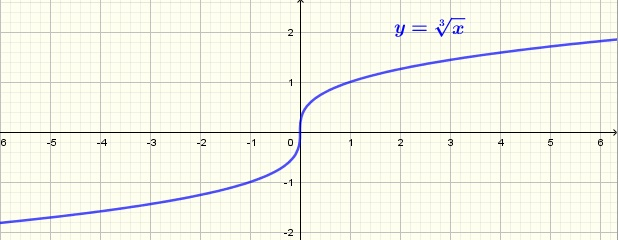# Cube Root Calculator

 

An online calculator to calculate the cube root of a real number. The definition and formulas for the cube roots of a number are given below.

## Use of Cube Root Calculator

1 - Enter a real number x (-5.5 is the default value) in decimal form and the number of decimal places (positive integer) desired and press "Calculate Cube Root".

 x = -5.5 Decimal Places = 3 Real Root = Complex Root (1) = + i Complex Root (2) = + i

## Real Cube Root of a Real Number

A number y is the cube root of a number x if $$y^3 = x$$.
Numerical examples
$$1$$ is the cube root of 1 because $$1^3 = 1$$
$$2$$ is the cube root of 8 because $$2^3 = 8$$
$$- 10$$ is the cube root of - 1000 because $$(- 10)^3 = - 1000$$
The cube root function notation is as follows

$$y = \sqrtx$$

The graph of the cube root functions is shown below.## Complex Cube Root of a Real Number

Up to this point, the cube root is a real number. If we allow the cube root to be a complex number, then according to Demoivre's theorem there are three cube roots, one real and two complex conjugate, for any non zero real x and these roots are:
one real root given by
$$y_1 = \sqrtx$$
two complex conjugate given by
$$y_2 = \sqrtx ( - \dfrac{1}{2} + i \dfrac{\sqrt3}{2})$$
$$y_3 = \sqrtx ( - \dfrac{1}{2} - i \dfrac{\sqrt3}{2})$$
where $$i$$ is the imaginary unit such that $$i = \sqrt{-1}$$.
Example
The three cube roots of - 27 are
$$y_1 = \sqrt{-27} = - 3$$
$$y_2 = \sqrt{-27} ( - \dfrac{1}{2} + i \dfrac{\sqrt3}{2}) = \dfrac{3}{2} - \dfrac{3\sqrt3}{2}$$
$$y_3 = \sqrt{-27} ( - \dfrac{1}{2} - i \dfrac{\sqrt3}{2}) = \dfrac{3}{2} + \dfrac{3\sqrt3}{2}$$